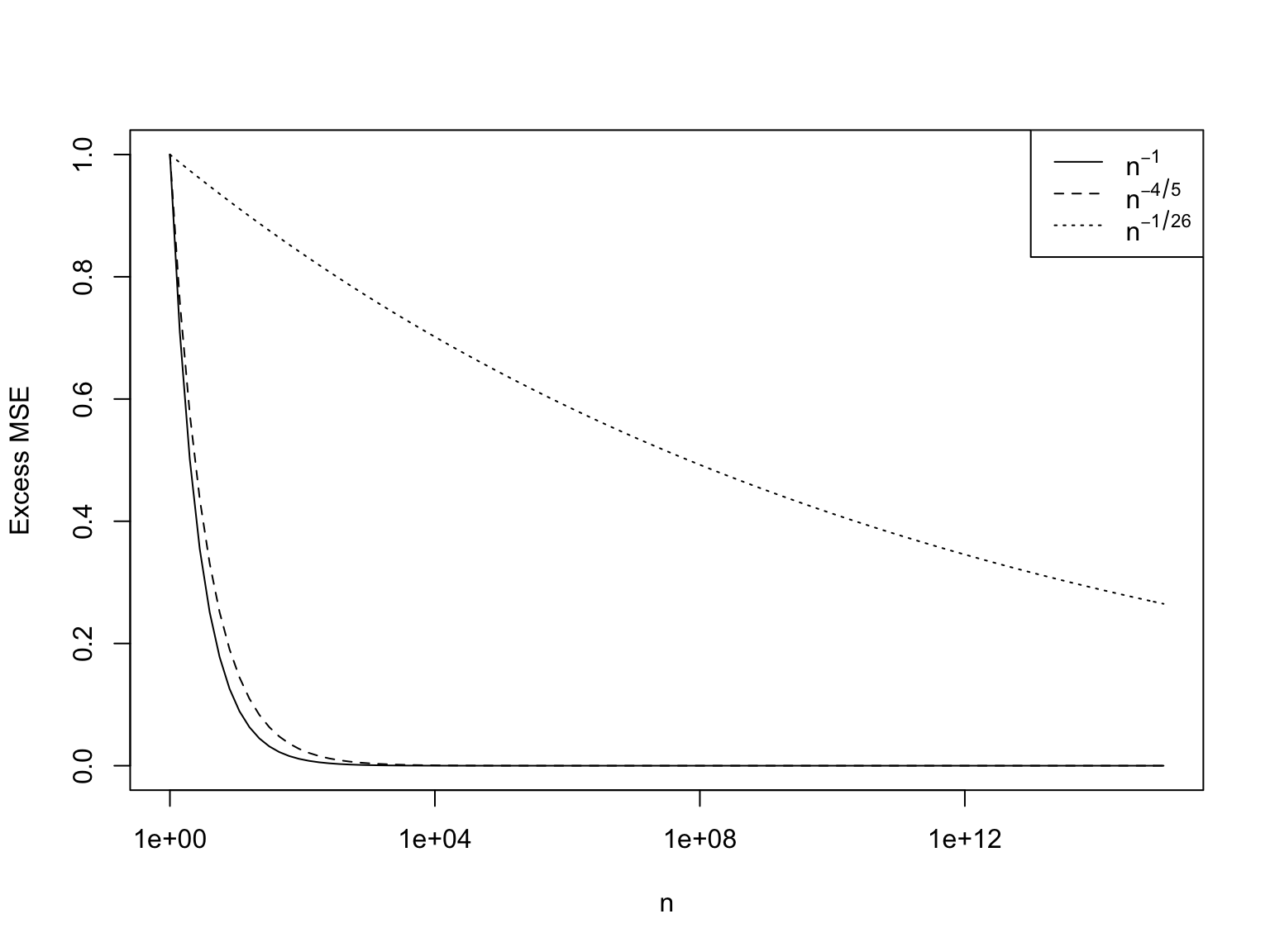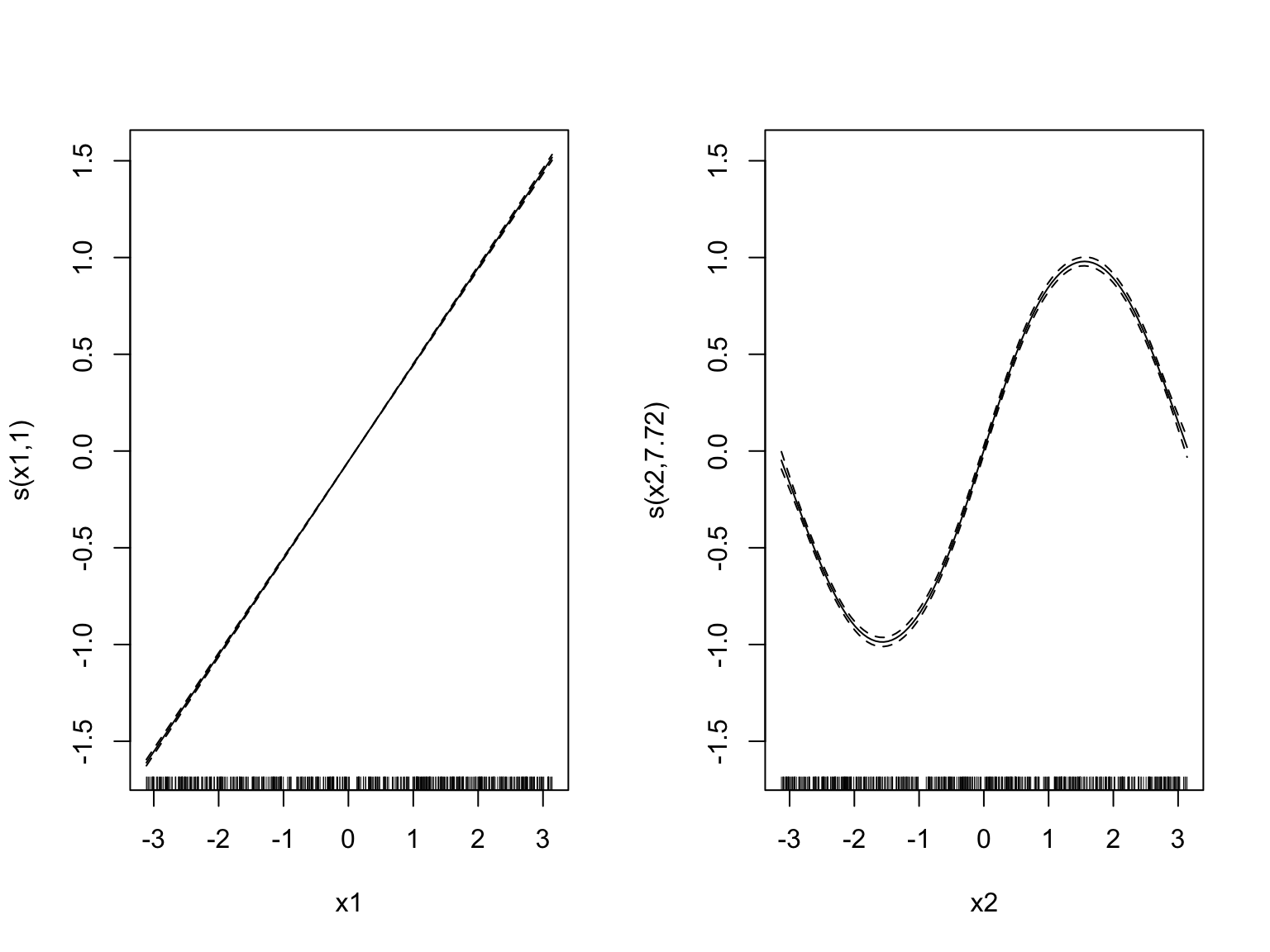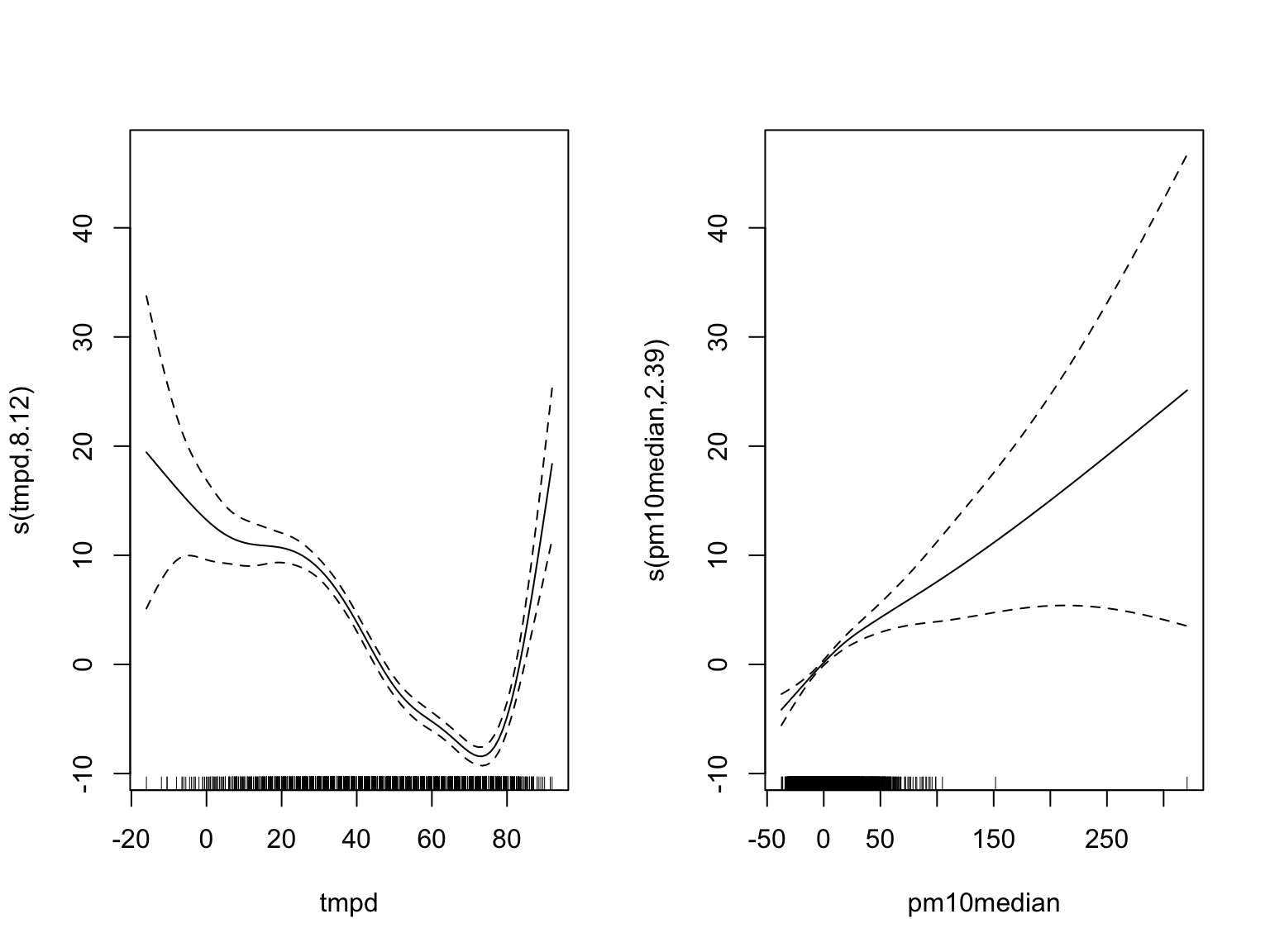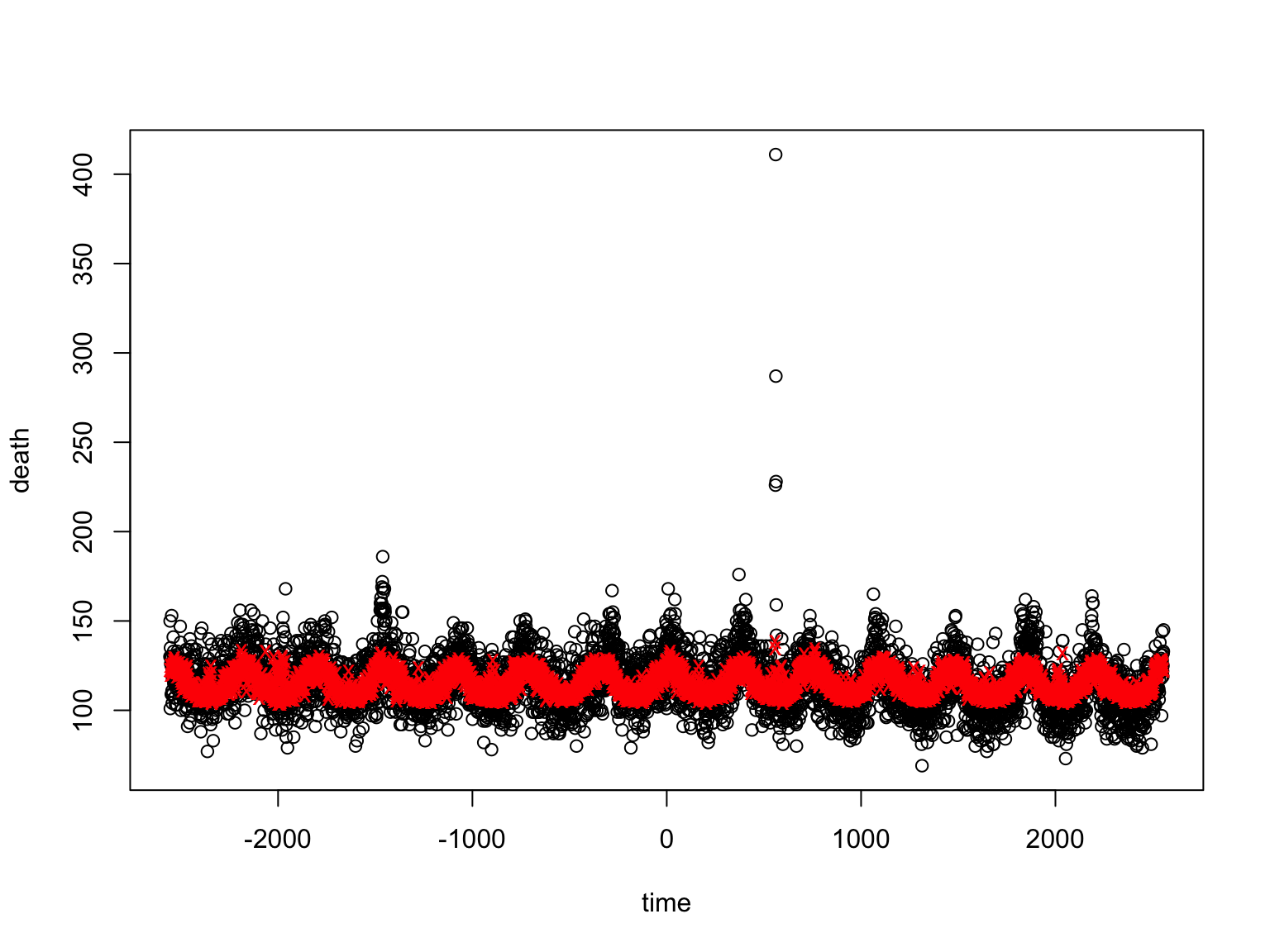19 February 2019

$\newcommand{\TrueRegFunc}{\mu} \newcommand{\EstRegFunc}{\widehat{\mu}} \newcommand{\Expect}{\mathbb{E}\left[ #1 \right]} \newcommand{\Var}{\mathbb{V}\left[ #1 \right]} \DeclareMathOperator*{\argmin}{argmin} % thanks, wikipedia!$

# Previously:

• We want to predict $$Y$$ from $$X$$ with small MSE, $$\Expect{(Y-m(X))^2}$$
• Optimum would be $$\TrueRegFunc(x) = \Expect{Y|X=x}$$; then $$Y=\TrueRegFunc(X)+\epsilon$$ with $$\Expect{\epsilon|X=x}=0$$ for all $$x$$
• $$\sigma^2 \equiv \Expect{(Y-\mu(X))^2} > 0$$ (generally)
• We try to learn/estimate $$\TrueRegFunc$$ from data $$(x_1, y_1), \ldots (x_n, y_n)$$, getting an approximation $$\EstRegFunc$$
• Linear smoothers: $\EstRegFunc(x_0) = \sum_{i=1}^{n}{y_i w(x_0, x_i)}$

# Previously (cont’d):

• Kernel smoothing, 1D: $\EstRegFunc(x_0) = \sum_{i=1}^{n}{y_i \frac{K(\frac{x_0-x_i}{h})}{\sum_{k=1}^{n}{K(\frac{x_0-x_k}{h})}}}$
• Kernel smoothing, $$p$$-dimensional: $\EstRegFunc(x_0) = \sum_{i=1}^{n}{y_i \frac{\prod_{j=1}^{p}{K(\frac{x_{0j}-x_{ij}}{h_j})}}{\sum_{k=1}^{n}{\prod_{j=1}^{p}{K(\frac{x_{0j}-x_{kj}}{h_j})}}}}$
• Bias of kernel smoothing is $$O(h^2)$$ in any dimension
• Variance of kernel smoothing is $$O(n^{-1} h^{-p})$$ in $$p$$ dimensions

# EXERCISE

• Bias is $$O(h^2)$$, variance is $$O(n^{-1} h^{-p})$$
• MSE of kernel smoothing is $MSE(h) = \sigma^2 + b h^4 + v n^{-1}h^{-p}$
• (plus negligible remainders)
1. What’s the best bandwidth? How does it change with $$n$$ and $$p$$?
2. Does the bias at the best bandwidth $$\rightarrow 0$$ as $$n\rightarrow\infty$$?
3. Does the variance at the best bandwidth $$\rightarrow 0$$ as $$n\rightarrow\infty$$?
4. Does $$\EstRegFunc \rightarrow \TrueRegFunc$$ as $$n\rightarrow\infty$$?

# SOLUTION

#### Part 1:

$\begin{eqnarray} \left.\frac{dMSE}{dh}\right|_{h=h_{opt}} & = & 0 = 0 + 3bh_{opt}^3 - \frac{vp}{n}h_{opt}^{-p-1}\\ h_{opt}^{4+p} & = & \frac{vp}{3b}n^{-1}\\ h_{opt} & = & \left(\frac{vp}{3b}\right)^{1/(4+p)} n^{-\frac{1}{4+p}}\\ h_{opt} & = & O(n^{-\frac{1}{4+p}}) \end{eqnarray}$

#### Part 2:

$\begin{eqnarray} \mathrm{bias} & = & O(h_{opt}^2)\\ & = & O(n^{-\frac{2}{4+p}}) \rightarrow 0 \end{eqnarray}$

#### Part 3:

$\begin{eqnarray} \mathrm{variance} & = & O(n^{-1} h_{opt}^{-p})\\ & = & O(n^{-1} n^{-\frac{p}{4+p}})\\ & = & O(n^{-\frac{4}{4+p}}) \rightarrow 0 \end{eqnarray}$

#### Part 4:

Yes, if bias and variance both $$\rightarrow 0$$, then the estimate must converge on the truth

# Kernel regression is universally consistent

• In any dimension, as $$n\rightarrow \infty$$, the bias of $$\EstRegFunc$$ $$\rightarrow 0$$ and the variance $$\rightarrow 0$$, so $\EstRegFunc \rightarrow \TrueRegFunc$
• Kernel regression is universally consistent
• At least if $$\TrueRegFunc$$ is smooth enough for a 2nd-order Taylor series

# Kernel regression can converge very slowly

• The MSE at the best bandwidth is $MSE = \sigma^2+O(h_{opt}^4) = \sigma^2 + O(n^{-\frac{4}{4+p}})$
• So MSE approaches that of $$\TrueRegFunc$$
• BUT we need exponentially more data as the dimension grows
• With $$n={10}^{12}$$ and $$p=1$$, $$n^{-4/5} = 2.5\times 10^{-10}$$
• With $$n={10}^{12}$$ and $$p=100$$, $$n^{-4/(104)} = 0.35$$

# Kernel regression can converge very slowly# The problem isn’t kernel regression

• $$k$$-nearest neighbors is also universally consistent, and also has the same rate of convergence
• So do splines, trees, random forests, deep neural networks, …
• In fact, this is the best possible rate for any universally-consistent method
• You can converge faster for some $$\TrueRegFunc$$, if you don’t converge at all for others
• This — exponentially slow convergence as $$p$$ grows — is the curse of dimensionality

# Lifting the curse

• Impose special structure on $$\TrueRegFunc$$, either because:
• We believe it’s true, or
• We’re willing to make that approximation
• Extreme example: Only use linear functions
• If $$\TrueRegFunc$$ is linear, then $$MSE = \sigma^2 + O(p/n)$$
• If $$\TrueRegFunc$$ isn’t linear, then $$MSE = \sigma^2 + (\mathrm{bias\ of\ best\ linear\ model})^2 + O(p/n) = \sigma^2 + O(1)$$
• Can we do something less extreme than linear models?

• Linear model: $Y = \beta_0 + \sum_{j=1}^{p}{\beta_j x_j} + \epsilon$
• Additive model: $Y = \alpha + \sum_{j=1}^{p}{f_j(x_j)} + \epsilon$
• Every linear model is additive, $$f_j(x_j) = \beta_j x_j$$
• Not every additive model is linear, e.g, $$x_1 + \sin{(x_2)} + \log{(1+x_3)}$$
• The $$f_j$$ are called partial response functions
• To get total response (= prediction for $$Y$$), add up all the partial responses, plus $$\alpha$$
• All else being equal, if you change $$x_j$$ to $$x_j^{\prime}$$, the predicted $$Y$$ changes by $$f_j(x_j^{\prime}) - f_j(x_j)$$
• Like transforming your predictors in a linear model

# So how do we estimate an additive model?

• Re-arrange terms $f_j(x_j) + \epsilon = Y - (\alpha + \sum_{k \neq j}{f_k(x_k)})$
• If we knew all the other functions, we could estimate $$f_j$$ by 1D smoothing

#### Backfitting (or Gauss-Seidel)

1. Start with $$\alpha = \overline{y}$$, and $$f_1 = f_2 \ldots = f_p = 0$$
2. For $$j \in 1:p$$:
1. $$r_i \leftarrow y_i - (\alpha + \sum_{k\neq j}{f_k(x_{ik})})$$ (partial residual)
2. Use your favorite smoother to regress $$r$$’s on $$x_j$$ (1D smoothing), get function $$s_j$$
3. $$f_j \leftarrow s_j - \overline{s_j}$$ (keep functions centered at 0)
3. Repeat (1) until things stop changing

# In R: mgcv

• Several packages available, older gam and newer, nicer mgcv
• Main function is called gam (Generalized Additive Model)
• We’ll get to the “generalized” in a few weeks
• Syntax is very like lm but can tell it to smooth variables:
library(mgcv)
x <- matrix(runif(1000, min=-pi, max=pi), ncol=2)
y <- 0.5*x[,1]+sin(x[,2])+rnorm(n=nrow(x), sd=0.1)
df <- data.frame(y=y, x1=x[,1], x2=x[,2])
babys.first.am <- gam(y ~ s(x1)+s(x2), data=df)
par(mfrow=c(1,2)); plot(babys.first.am)# s()

• People often read s() as “smooth”, but it really means “spline”
• A smoothing spline is a curve balancing fit to data against wiggliness: $s_{\lambda} = \argmin_{m}{\frac{1}{n}\sum_{i=1}^{n}{(y_i - m(x_i))^2} + \lambda\int{\left(\frac{d^2 m}{dx^2}(x)\right)^2 dx}}$
• Solution for each $$\lambda$$ is a smooth, piecewise-cubic polynomial
• $$s_{\lambda}$$ is linear in $$\mathbf{y}$$ (surprisingly)
• Polynomials are fast to calculate and estimate
• $$\lambda\rightarrow 0$$ gives interpolation; $$\lambda\rightarrow\infty$$ gives a linear model
• (There are also other kinds of spline)
• gam() knows about lots of other smoother techniques
• te(), ti(), etc., etc., etc.
• Mostly about how to handle interactions
• See textbook

# Methods for mgcv::gam

• predict: Works just like with lm (or npreg for that matter), see help(predict.gam) for details
• fitted, residuals: Work just like with lm
• plot: We just saw this; many options, some described in text, see help(plot.gam) for details
• coefficients: Mostly coefficients of the spline polynomials — NOT INTERPRETABLE
• Can include linear terms, e.g., gam(y ~ x1+s(x2))
• Can include interaction terms, e.g., gam(y ~ s(x1, x2))

# A small real-data example

library(gamair)
data(chicago)
chicago.am <- gam(death ~ s(tmpd) + s(pm10median), data=chicago)

# A small real-data example (cont’d)

par(mfrow=c(1,2)); plot(chicago.am)# A small real-data example (cont’d)

# Handling missing values by padding in NAs:
am.fits <- predict(chicago.am, newdata=chicago, na.action=na.pass)
plot(death ~ time, data=chicago)
points(chicago\$time, am.fits, pch="x", col="red")# But what about the curse?

• If $$\TrueRegFunc$$ is additive, then $MSE = \sigma^2 + O(p n^{-4/5})$ which is pretty good
• If $$\TrueRegFunc$$ is not additive, then $MSE = \sigma^2 + (\mathrm{bias\ of\ best\ additive\ model})^2 + O(pn^{-4/5})$
• This is still better, as $$n\rightarrow\infty$$, than linear models

# Summing up

• Estimating regression functions in high dimensions is intrinsically hard
• We need either a lot of data OR special assumptions about the function
• Additive models are an easy step beyond linear models with a lot of advantages
• Get used to typing gam rather than lm